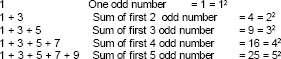# Square Root and Cube Root

Square Root and Cube Root: In mathematics, the square root of a number x is a number y such that y2 = x; in other words, a number y whose square (the result of multiplying the number by itself, or y ⋅ y) is x. For example, 4 and −4 are square roots of 16 because 42 = (−4)2 = 16. Every non-negative real number x has a unique non-negative square root, called the principal square root, which is denoted by √x, where the symbol √  is called the radical sign or radix. For example, the principal square root of 9 is 3, which is denoted by √9 = 3, because 32 = 3 ⋅ 3 = 9 and 3 is non-negative.

1.   When we multiply a number by itself three times, the product so obtained is called the perfect cube of that number.
2. There are only 10 perfect cubes from 1 to 1000.
3. Cubes of even numbers are even and those of odd numbers are odd
4. The cube of a negative number is always negative.
5. If the digit in one�fs place of a number is 0, 1, 4, 5, 6 or 9, then its cube will end in the same digit.
6. If the digit in one�fs place of a number is 2, then its cube will end in 8 and vice-versa.
7. If the digit in one�fs place of a number is 3, then its cube will end in 7 and vice-versa.
8. If the prime factor of a number can not be made into groups of 3, it is not a perfect square.
9. The symbol 3�� denotes the cube root of a number.

•  Square of a number.
If a natural number m can be expressed as n2 (where n is a natural number), then m is the square root or perfect square.
i.e. if m = n2          (m, n – natural numbers)
E.g. 81 = 3 × 3 × 3 × 3
= 32 × 32 = (3 × 3)2 = 92
Hence, 9 is the square root of 81.

•  Properties of Square Root.
Below is the table that has squares of numbers from 1 to 10.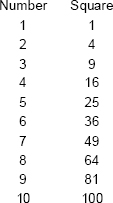If we see the above results carefully, we can conclude that numbers ending with 0, 1, 4, 5, 6, or 9 at units place are perfect squares None of these end with 2, 3, 7 or 8, So numbers that end with 2, 3, 7, 8 are not perfect squares.
Thus, numbers like 122, 457, 183, 928 are not perfect squares.

• One’s digit in square of a number.
(1). The ones digit in the square of number can be determined if the ones digit of the number is known.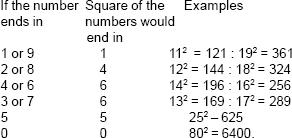(2). The number of zeros at the end of a perfect square is always even and double the number of zeros at the end of the number
E.g.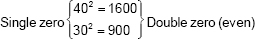Double zero {7002 = 490000} four zero (even)
(3). The square of an even number is always an even number and square of an odd number is always an odd number.
E.g.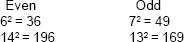• Interesting patterns of Square Root.

Number between square numbers
There are ‘2a’ non perfect square numbers between the square of two Consecutive natural numbers n + (n + 1)
Between 22 = 4 & 32 = 9 → 5, 6, 7, 8
2 × 2 = 4 non square numbers
Between
32 = 9 & 42 = 16 → 10, 11, 12, 13, 14, 15
2 × 3 = 6 non square numbers.